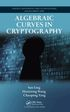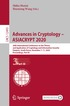Fler böcker inom
Format
Inbunden (Hardback)
Språk
Engelska
Antal sidor
340
Utgivningsdatum
2013-07-05
Förlag
Chapman & Hall/CRC
Illustrationer
4 Tables, black and white; 15 Illustrations, black and white
Dimensioner
241 x 163 x 22 mm
Vikt
617 g
Antal komponenter
1
ISBN
9781420079463

### Du kanske gillar# Algebraic Curves in Cryptography

Inbunden Engelska, 2013-07-05
889
Skickas inom 7-10 vardagar.
Gratis frakt inom Sverige över 159 kr för privatpersoner.
Finns även som
• Skickas inom 10-15 vardagar
749
• Tillfälligt slut
579
The reach of algebraic curves in cryptography goes far beyond elliptic curve or public key cryptography yet these other application areas have not been systematically covered in the literature. Addressing this gap, Algebraic Curves in Cryptography explores the rich uses of algebraic curves in a range of cryptographic applications, such as secret sharing, frameproof codes, and broadcast encryption. Suitable for researchers and graduate students in mathematics and computer science, this self-contained book is one of the first to focus on many topics in cryptography involving algebraic curves. After supplying the necessary background on algebraic curves, the authors discuss error-correcting codes, including algebraic geometry codes, and provide an introduction to elliptic curves. Each chapter in the remainder of the book deals with a selected topic in cryptography (other than elliptic curve cryptography). The topics covered include secret sharing schemes, authentication codes, frameproof codes, key distribution schemes, broadcast encryption, and sequences. Chapters begin with introductory material before featuring the application of algebraic curves.

## Passar bra ihop

1.2. +
3.De som köpt den här boken har ofta också köpt Advances in Cryptology - ASIACRYPT 2020 av Shiho Moriai, Huaxiong Wang (häftad).

## Kundrecensioner

Har du läst boken? Sätt ditt betyg »

## Recensioner i media

"This is a self-contained book intended for researchers and graduate students in mathematics and computer science interested in different topics in cryptography involving algebraic curves ... The authors of this book make an exhaustive review on some other topics where algebraic curves, mainly in higher genus, are important as well." -Zentralblatt MATH 1282 "The book is written in a user-friendly style, with good coverage of the background, many examples, and a detailed bibliography of over 180 items. It is mainly directed towards graduate students and researchers, but some parts of the book are even accessible for advanced undergraduate students. The book is highly recommended for readers interested in the manifold applications of algebraic curves over finite fields." -Harald Niederreiter, Mathematical Reviews, March 2014 "The book is filled with examples to illustrate the various constructions and, assuming a basic knowledge of combinatorics and algebraic geometry, it is almost self-contained." -Felipe Zaldivar, MAA Reviews, September 2013

## Övrig information

San Ling is a professor in the Division of Mathematical Sciences, School of Physical and Mathematical Sciences at Nanyang Technological University. He received a PhD in mathematics from the University of California, Berkeley. His research interests include the arithmetic of modular curves and application of number theory to combinatorial designs, coding theory, cryptography, and sequences. Huaxiong Wang is an associate professor in the Division of Mathematical Sciences at Nanyang Technological University. He is also an honorary fellow at Macquarie University. He received a PhD in mathematics from the University of Haifa and a PhD in computer science from the University of Wollongong, Australia. His research interests include cryptography, information security, coding theory, combinatorics, and theoretical computer science. Chaoping Xing is a professor at Nanyang Technological University. He received a PhD from the University of Science and Technology of China. His research focuses on the areas of algebraic curves over finite fields, coding theory, cryptography, and quasi-Monte Carlo methods.

## Innehållsförteckning

Introduction to Algebraic Curves Plane Curves Algebraic Curves and Their Function Fields Smooth Curves Riemann-Roch Theorem Rational Points and Zeta Functions Introduction to Error-Correcting Codes Introduction Linear Codes Bounds Algebraic Geometry Codes Asymptotic Behavior of Codes Elliptic Curves and Their Applications to Cryptography Basic Introduction Maps between Elliptic Curves The Group E(Fq) and Its Torsion Subgroups Computational Considerations on Elliptic Curves Pairings on an Elliptic Curve Elliptic Curve Cryptography Secret Sharing Schemes The Shamir Threshold Scheme Other Threshold Schemes General Secret Sharing Schemes Information Rate Quasi-Perfect Secret Sharing Schemes Linear Secret Sharing Schemes Multiplicative Linear Secret Sharing Schemes Secret Sharing from Error-Correcting Codes Secret Sharing from Algebraic Geometry Codes Authentication Codes Authentication Codes Bounds of A-Codes A-Codes and Error-Correcting Codes Universal Hash Families and A-Codes A-Codes from Algebraic Curves Linear Authentication Codes Frameproof Codes Introduction Constructions of Frameproof Codes without Algebraic Geometry Asymptotic Bounds and Constructions from Algebraic Geometry Improvements to the Asymptotic Bound Key Distribution Schemes Key Predistribution Key Predistribution Schemes with Optimal Information Rates Linear Key Predistribution Schemes Key Predistribution Schemes from Algebraic Geometry Key Predistribution Schemes from Cover-Free Families Perfect Hash Families and Algebraic Geometry Broadcast Encryption and Multicast Security One-Time Broadcast Encryption Multicast Re-Keying Schemes Re-Keying Schemes with Dynamic Group Controllers Some Applications from Algebraic Geometry Sequences Introduction Linear Feedback Shift Register Sequences Constructions of Almost Perfect Sequences Constructions of Multisequences Sequences with Low Correlation and Large Linear Complexity Bibliography Index